﻿• 价格透明
• 信息保密
• 进度掌控
• 售后无忧# Android自动生成Shape资源文件（下）

系列文章目录

 第一章：Android自动生成代码，可视化脚手架，将大大提高开发效率 第二章：Android自动生成代码，可视化脚手架之环境搭建 第三章：Android自动生成代码，可视化脚手架之基础信息配置 第四章：Android自动生成Shape资源文件，迈出可视化脚手架第一步（上）

https://github.com/AbnerMing888/AndroidShortcutTools

## 一、页面绘制及保存到drawable文件夹下。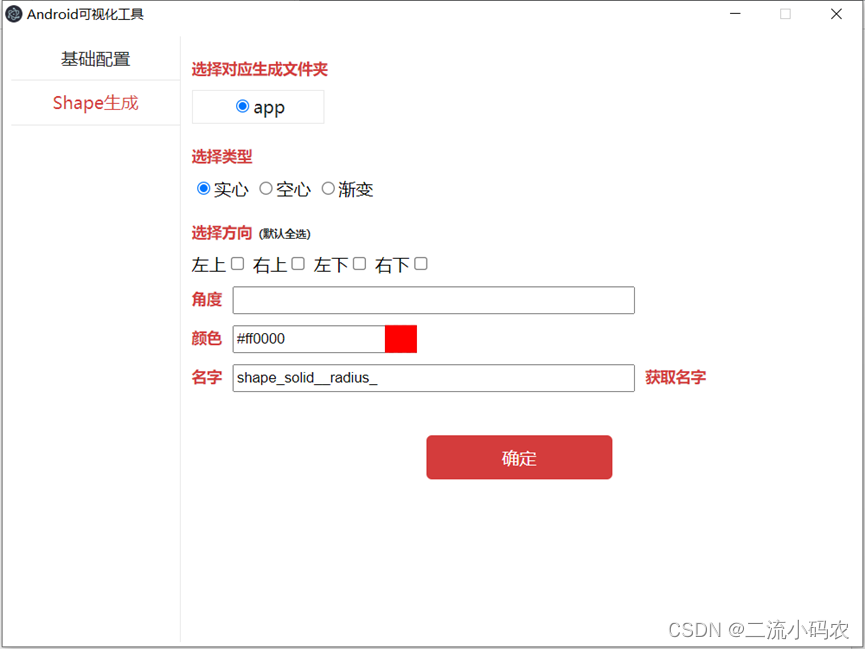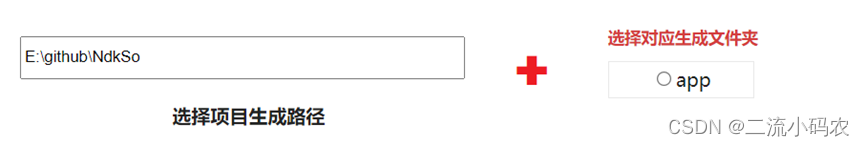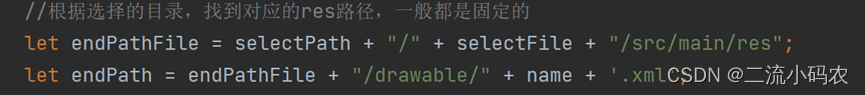``````//先判断drawable文件是否存在，不存在去创建
if (err) {
return
}
var booDrawable = false;
files.forEach(function (item) {
if ("drawable" === item) {
booDrawable = true;
}
});
//存在
if (booDrawable) {
writeDrawable(endPath, endShapeText);
} else {
//不存在，创建
fs.mkdir(endPathFile + "/drawable", function (err) {
if (err) {
return;
}
writeDrawable(endPath, endShapeText);
});
}
});
``````

``````//检测选择的是否是一个Android项目，通过是否包含app，gradle，settings.gradle，当然也可以判断其他
if (item === "app" || item === "gradle" || item === "settings.gradle") {
numAndroid++;
}
``````

## 二、根据对应模板代码和UI视图选择，生成对应的资源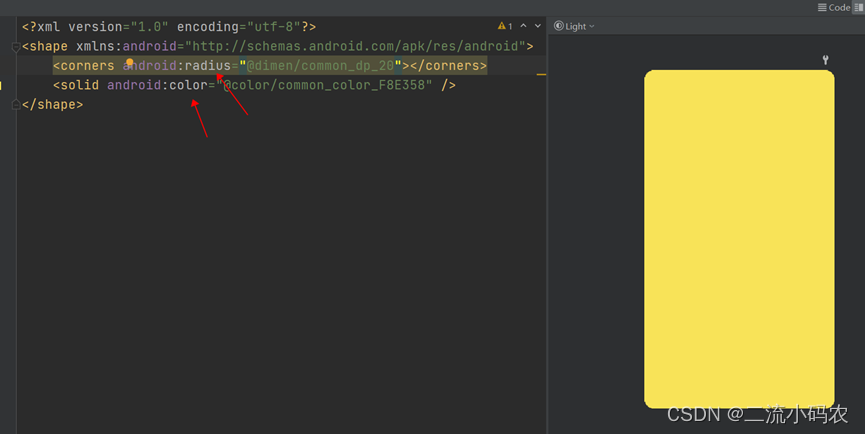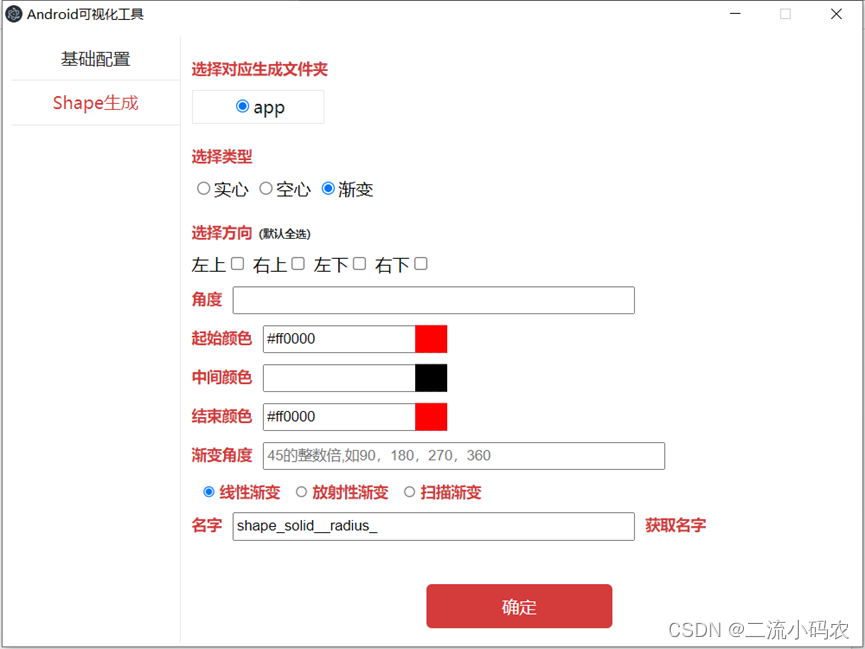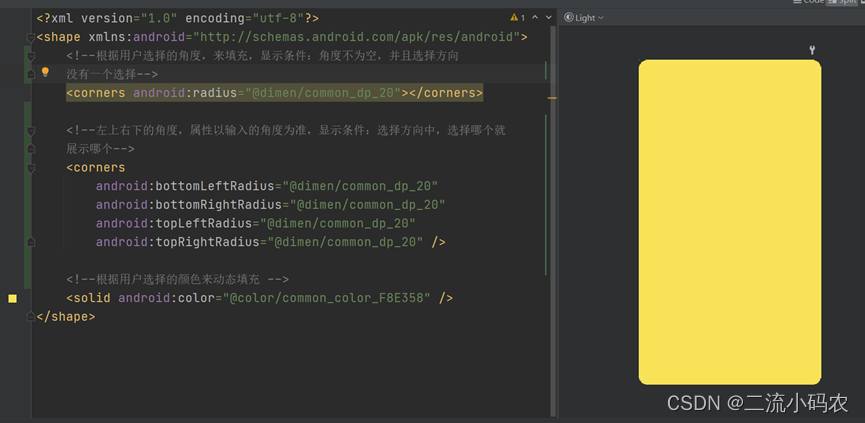``````//获取实心的代码
var content = "<?xml version=\"1.0\" encoding=\"utf-8\"?>\n" +
"<shape xmlns:android=\"http://schemas.android.com/apk/res/android\">\n";

//不为空
if (radius != "" && radius != null && selectDp != null && selectDp != "") {
//取出默认的dp配置前缀
} else {
}

if (radius != null && (checkText == "" || checkText == "0123")) {
//全部
} else {
content = content + "    <corners\n";
if (checkText.indexOf("0") != -1) {
}
if (checkText.indexOf("1") != -1) {
}
if (checkText.indexOf("2") != -1) {
}
if (checkText.indexOf("3") != -1) {
}
content = content + "        />\n";
}
//基础信息color不为空，就追加前缀
color = getEndColor(color);
content = content + "    <solid android:color=\"" + color + "\" />\n";
content = content + "</shape>";
return content;
}
``````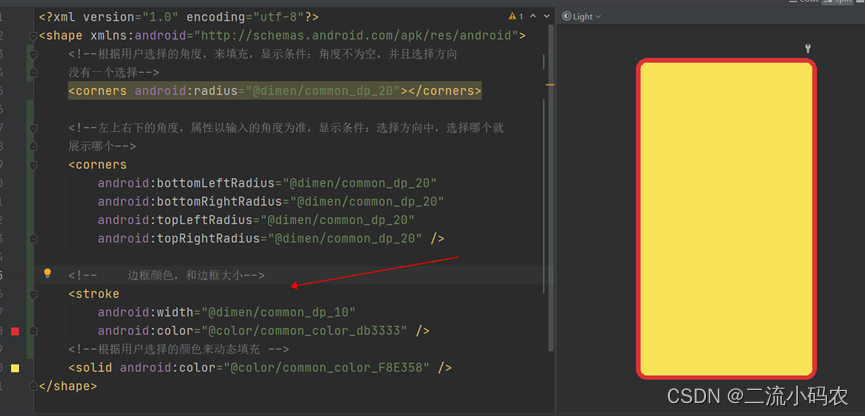``````//获取空心也就是带有边框的代码
function getStrokeText(radius, solid, react, reactSize, checkText) {
//不为空
if (radius != "" && radius != null && selectDp != null && selectDp != "") {
//取出默认的dp配置前缀
} else {
}

if (reactSize != "" && reactSize != null && selectDp != null && selectDp != "") {
//取出默认的dp配置前缀
reactSize = selectDp + reactSize;
} else {
reactSize = reactSize + "dp";
}
//边框颜色
//基础信息color不为空，就追加前缀
react = getEndColor(react);
var content = "<?xml version=\"1.0\" encoding=\"utf-8\"?>\n" +
"<shape xmlns:android=\"http://schemas.android.com/apk/res/android\"\n" +
"    >\n";
content = content + "    <stroke\n" +
"        android:width=\"" + reactSize + "\"\n" +
"        android:color=\"" + react + "\" />\n";
if (radius != null && (checkText == "" || checkText == "0123")) {
//全部
content = content + "    <corners android:radius=\"" + radius + "\" />\n";
} else {

content = content + "    <corners \n";
if (checkText.indexOf("0") != -1) {
}
if (checkText.indexOf("1") != -1) {
}
if (checkText.indexOf("2") != -1) {
}
if (checkText.indexOf("3") != -1) {
}
content = content + "        />\n";
}

solid = getEndColor(solid);

content = content + " <solid android:color=\"" + solid + "\"/>\n";
content = content + "</shape>"
return content;
}
``````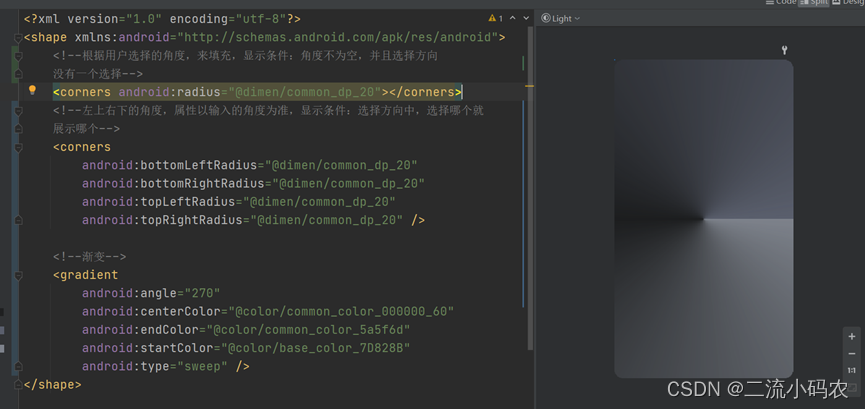代码实现：

``````//获取渐变
//渐变
var inputReactStartColor = \$(".input_react_start_color").val();//起始颜色
var inputReactCenterColor = \$(".input_react_center_color").val();//中间颜色
var inputReactEndColor = \$(".input_react_end_color").val();//结束颜色

inputReactStartColor = getEndColor(inputReactStartColor);
inputReactCenterColor = getEndColor(inputReactCenterColor);
inputReactEndColor = getEndColor(inputReactEndColor);

showToast("请输入渐变角度");
return "";
}
var sgType;
sgType = "linear";
} else if (shapeGradientType == 1) {
} else {
sgType = "sweep";
}
var gradient = "<?xml version=\"1.0\" encoding=\"utf-8\"?>\n" +
"<shape xmlns:android=\"http://schemas.android.com/apk/res/android\">\n" +
"\n" +

if (inputReactCenterColor != null && inputReactCenterColor != "") {
}
"        android:startColor=\"" + inputReactStartColor + "\"\n" +
"        android:type=\"" + sgType + "\" />\n"
//不为空
//取出默认配置前缀，若不为空，就追加
if (selectDp != "" && selectDp != null) {
} else {
}
if (checkText == "" || checkText == "0123") {
//全部
} else {
if (checkText.indexOf("0") != -1) {
}
if (checkText.indexOf("1") != -1) {
}
if (checkText.indexOf("2") != -1) {
}
if (checkText.indexOf("3") != -1) {
}
}
}

}
``````

Shape的可视化生成就是如此的简单，好了各位老铁，下一篇，我们搞一个可视化的多渠道打包，一秒可生成N个渠道包，敬请期待！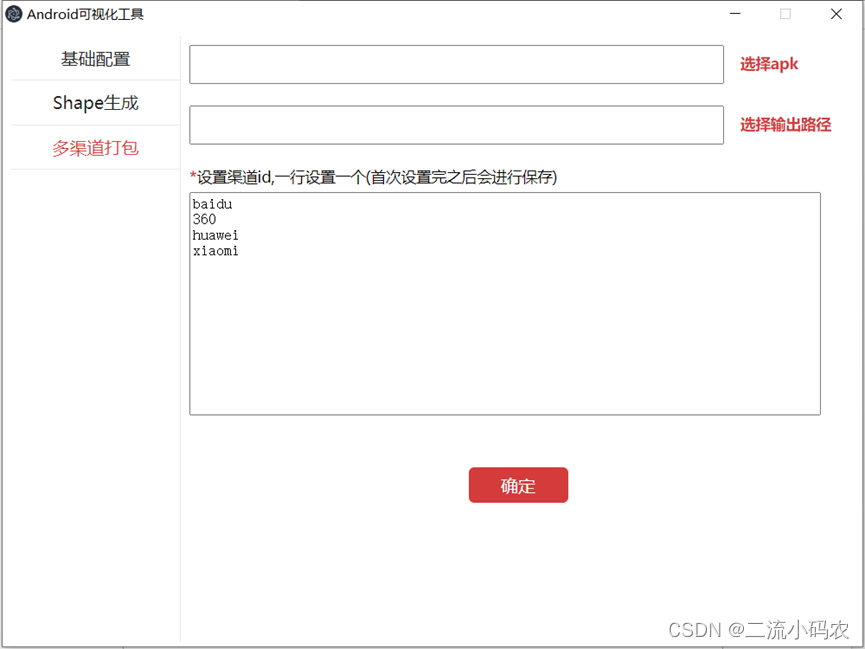### 低价透明### 金牌服务### 信息保密